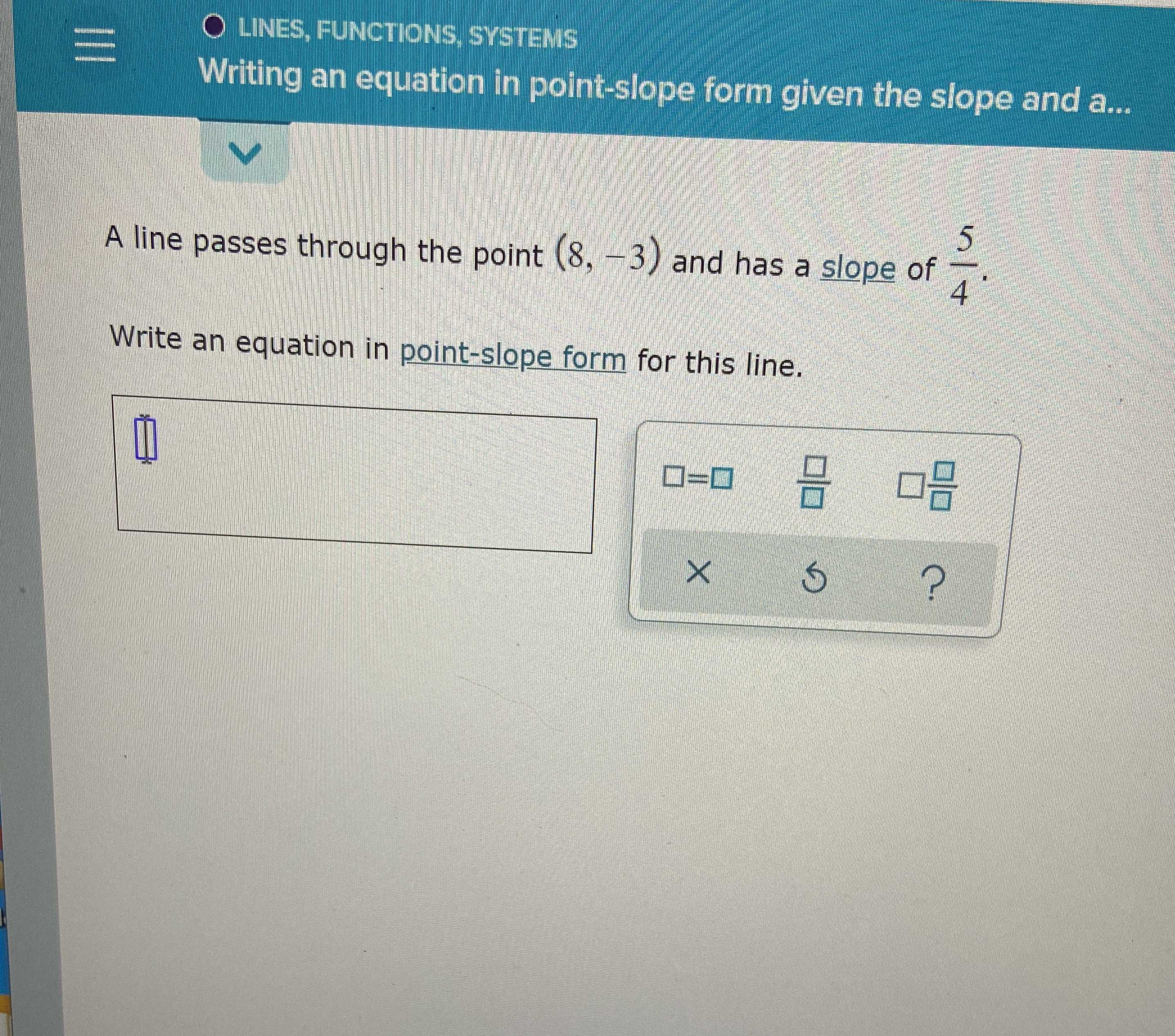### ¿Todavía tienes preguntas de matemáticas?

Pregunte a nuestros tutores expertos
Algebra
PreguntaA line passes through the point $$( 8 , - 3 )$$ and has a slope of $$\frac { 5 } { 4 }$$ . Write an equation in point-slope form for this line.

$$(y+ 3)= \frac{5}{4}(x- 8)$$